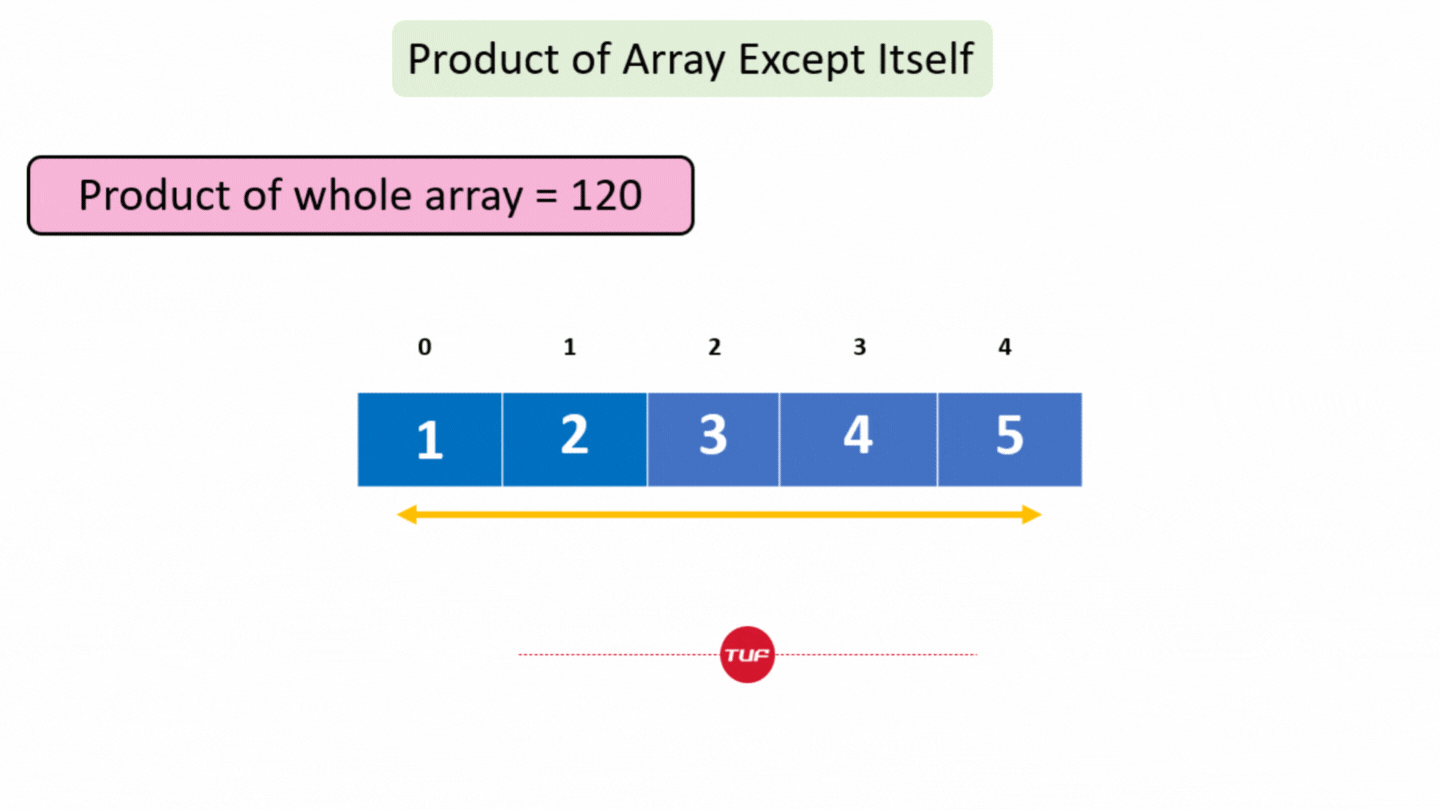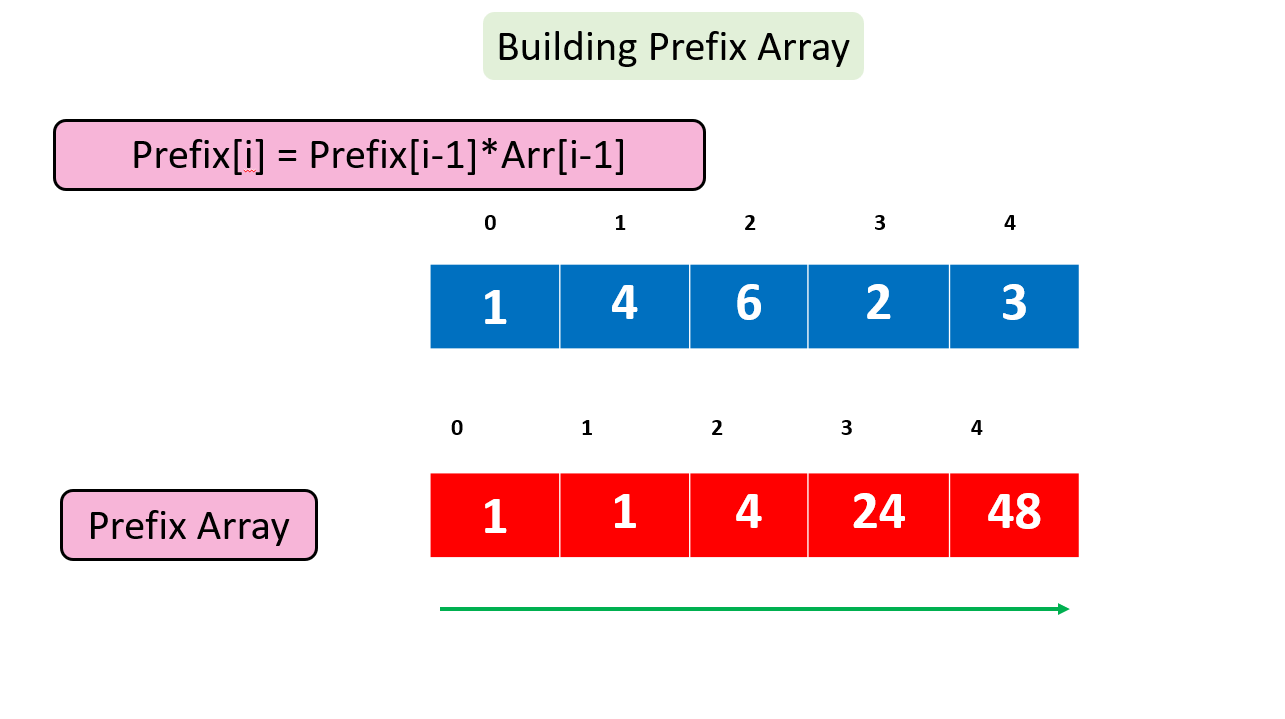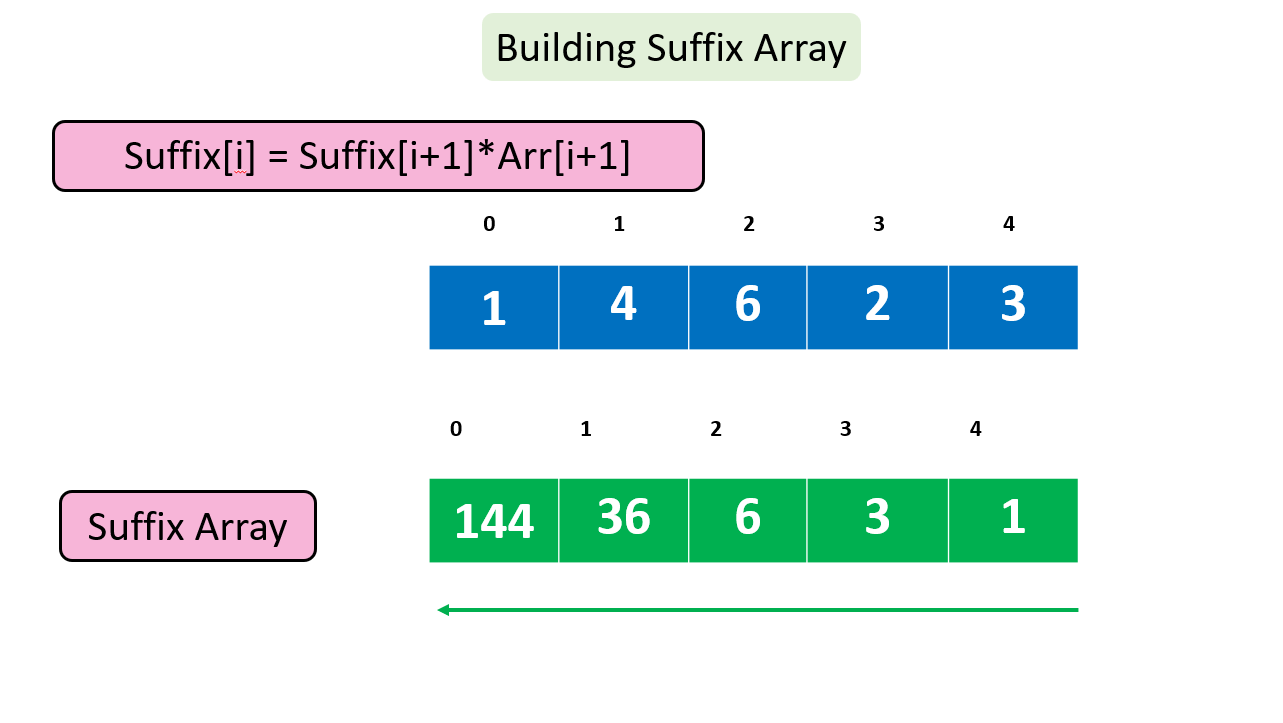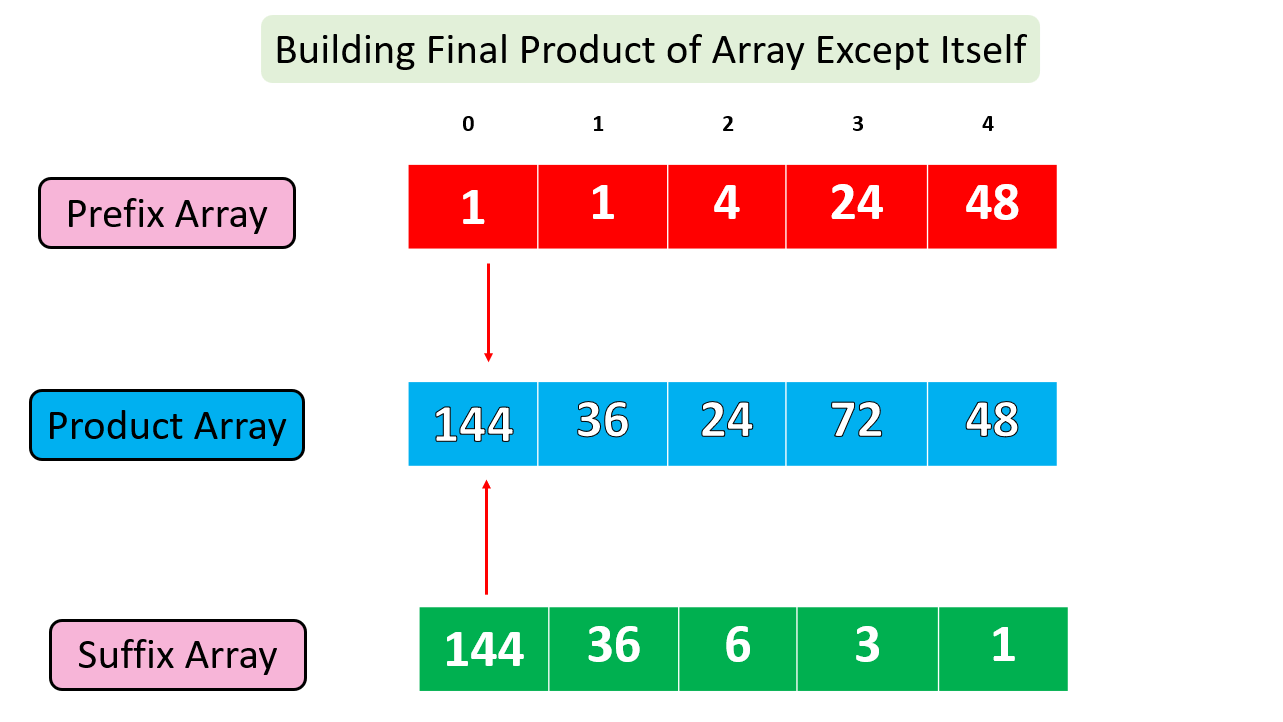# Product of Array Except Itself

Problem Statement: Given an array arr[] of integers, you need to return the product of given array elements except including the element itself.

NOTE – You should not use division operator ‘ / ’

Example:

```Input: N = 5, array[] = {1,2,3,4,5}
Output: 120 60 40 30 24
Explanation:
For 0th index, excluding 1 from product of whole array will give 120
For 1th index, excluding 2 from product of whole array will give 60
For 2nd index , excluding 3 from product of whole array will give 40
For 3rd index, excluding 4 from product of whole array will give 30
For 4th index , excluding 5 from product of whole array will give 24
```### Solution

Disclaimer: Don’t jump directly to the solution, try it out yourself first.

### Solution 1: Maintaining Prefix and Suffix Array

Approach:

In this approach, we will use two extra arrays and maintain prefix and suffix products up to the current index. Let’s understand using an array.

```Arr = { 1 , 4 , 6 , 2 , 3}       N=5
Prefix Arr ={ 1 , 1 , 4 , 24 , 48 }
Suffix Arr ={ 144 , 36 , 6 , 3 , 1 }
```

For obtaining Prefix Array, Start from the first Index and multiply element one by one and store it in an array. Similarly, for obtaining a Suffix Array, Start from the end and multiply elements one by one, and store them in an array. Now moving on to every index, take the product of prefix and suffix Array to get the product of the whole array except itself.Now constructing suffix ArrayUsing them to compute the product Array (Having product of the whole array except the element itself)Code:

## C++ Code

``````#include <bits/stdc++.h>
using namespace std;
// Function to find Product of Array Except Itself
void findproduct(int arr[], int product[],int n)
{
int prefix[n];
prefix=1; // since first element can have no prefix
for(int i=1;i<n;i++)
{
prefix[i]=prefix[i-1]*arr[i-1];
}
int suffix[n];
suffix[n-1]=1; // since last element can have no suffix
for(int i=n-2;i>=0;i--)
{
suffix[i]=suffix[i+1]*arr[i+1];
}
// Building Product Array
for(int i=0;i<n;i++)
{
product[i]=prefix[i]*suffix[i];
}
}
// Driver Code
int main()
{
int arr[] = { 1 , 4 , 6 , 2 , 3};
int n = sizeof(arr)/sizeof(arr);
int product[n];
findproduct(arr,product,n);

cout << "The product of array Except itself is: ";
for(int i=0;i<n;i++)
{
cout<<product[i]<<" , ";
}
cout<<endl;

return 0;
}
``````

Output:

The product of array Except itself is: 144, 36, 24, 72, 48,

Time Complexity: O(n)

Space Complexity: O(n) + O(n)

## Java Code

``````class Main
{
// Function to find Product of Array Except Itself
public static void findproduct(int arr[], int product[],int n)
{
int prefix[]=new int[n];
prefix=1; // since first element can have no prefix
for(int i=1;i<n;i++)
{
prefix[i]=prefix[i-1]*arr[i-1];
}
int suffix[]=new int[n];
suffix[n-1]=1; // since last element can have no suffix
for(int i=n-2;i>=0;i--)
{
suffix[i]=suffix[i+1]*arr[i+1];
}
// Building Product Array
for(int i=0;i<n;i++)
{
product[i]=prefix[i]*suffix[i];
}
}
// Driver Code
public static void main(String[] args)
{
int arr[] = { 1 , 4 , 6 , 2 , 3};
int n = arr.length;
int product[]=new int[n];
findproduct(arr,product,n);

System.out.print("The product of array Except itself is: ");
for(int i=0;i<n;i++)
{
System.out.print(product[i]+", ");
}
System.out.println();
}
}
``````

Output:

The product of array Except itself is: 144, 36, 24, 72, 48,

Time Complexity: O(n)

Space Complexity: O(n) + O(n)

Solution 2: It is just a further optimized version without using Suffix Array.

We will create a prefix Array and then after that instead of creating a suffix array separately, start from the end of the array and maintain a variable suffixproduct which will keep track of the suffix product up to the current index.

Code:

## C++ Code

``````#include <bits/stdc++.h>
using namespace std;
// Function to find Product of Array Except Itself
void findproduct(int arr[], int product[],int n)
{
int prefix[n];
prefix=1; // since first element can have no prefix
for(int i=1;i<n;i++)
{
prefix[i]=prefix[i-1]*arr[i-1];
}
int suffixproduct=1;
// Building Product array and maintaining suffix product
for(int i=n-1;i>=0;i--)
{
product[i]=suffixproduct*prefix[i];
suffixproduct*=arr[i];
}
}
// Driver Code
int main()
{
int arr[] = { 1 , 4 , 6 , 2 , 3};
int n = sizeof(arr)/sizeof(arr);
int product[n];
findproduct(arr,product,n);

cout << "The product of array Except itself is: ";
for(int i=0;i<n;i++)
{
cout<<product[i]<<", ";
}
cout<<endl;

return 0;
}
``````

Output:

The product of array Except itself is: 144, 36, 24, 72, 48,

Time Complexity: O(n)

Space Complexity: O(n)

## Java Code

``````class Main
{
// Function to find Product of Array Except Itself
public static void findproduct(int arr[], int product[],int n)
{
int prefix[]=new int[n];
prefix=1; // since first element can have no prefix
for(int i=1;i<n;i++)
{
prefix[i]=prefix[i-1]*arr[i-1];
}
int suffixproduct=1;
// Building Product Array
for(int i=n-1;i>=0;i--)
{
product[i]=suffixproduct*prefix[i];
suffixproduct*=arr[i];
}
}
// Driver Code
public static void main(String[] args)
{
int arr[] = { 1 , 4 , 6 , 2 , 3};
int n = arr.length;
int product[]=new int[n];
findproduct(arr,product,n);

System.out.print("The product of array Except itself is: ");
for(int i=0;i<n;i++)
{
System.out.print(product[i]+", ");
}
System.out.println();
}
}
``````

Output:

The product of array Except itself is: 144, 36, 24, 72, 48,

Time Complexity: O(n)

Space Complexity: O(n)

Special thanks to Gurmeet Singh for contributing to this article on takeUforward. If you also wish to share your knowledge with the takeUforward fam, please check out this article